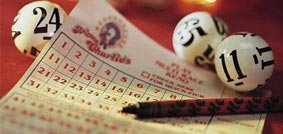# Keno Maths

Keno is a game of numbers, the outcome of which is determined entirely by chance. That is good news for anyone who is interested in exploring the mathematical side of the game, because it isn’t necessary to get bogged down in evaluating complicated theories about the physical properties of the gaming device or the skill level of the person who is playing. Instead, as long as the precise rules of the game being played are known, any sufficiently interested party can calculate the exact odds of almost any eventuality. That won’t help a player to win, of course, but it will give the number-cruncher a very good idea of what his or her chances are.

We don’t have space here to provide all of the formulae required to calculate every possible outcome in every possible variation of the game, but we can provide a fairly quick and easy mathematical formula that will enable you to find out what the odds of you succeeding are in a stated scenario. You will need a spreadsheet or scientific calculator with COMBIN function to actually use this formula, but it can be described quite simply without any calculation being required. The formula is as follows:

(W / T) x 100 = C%

Where:

W = The number of winning combinations
T = The total number of possible combinations
C = The Chance of Success, expressed as a percentage

## Using the Keno Formula

To use this formula, you will need to calculate W and T using a spreadsheet or scientific calculator using the COMBIN(n,s) function, where n=the numbers used and s=the numbers selected.

For example, if you wanted to calculate the chances of succeeding when picking 3 numbers in a game that draws 20 numbers from 80, your calculations would be:

W = COMBIN(20,3) = 1,140
T = COMBIN(80,3) = 82,160
1,140 divided by 82,160 = 0.0138
0.0138 x 100 = 1.38%

You can therefore conclude that the percentage chance of you catching all three of your numbers is 1.38%.

It is important to remember that this formula calculates the chance of you hitting all of your selections. Prizes can of course be won by matching fewer than all of your selections, especially when you play more than a handful of numbers to begin with.

Although the chance of a player winning the best possible prize by selecting the maximum quantity of numbers and matching them all is slim from a mathematical perspective, the fact that the chance exists is enough to make Keno a very popular game with those who like the idea of getting rich quick. The additional fact that consolation prizes exist for players who match fewer than all of their selections makes the game even more attractive.

### Play Keno NowPlay Keno Online